# Division with Remainders as Fractions

Division with Remainders as Fractions• The remainder is the amount left over after a division has shared an amount equally.
• Here we are sharing 5 carrots between 2 people with the division 5 ÷ 2.
• The people get 2 carrots each with 1 remaining.
• We can write 5 ÷ 2 = 2 remainder 1.
• We can divide this remainder of 1 by 2 to share it equally.
• 1 ÷ 2 is written as as a fraction as   1 / 2  .
• The two people each get 2   1 / 2   carrots each.
• 5 ÷ 2 = 2   1 / 2  .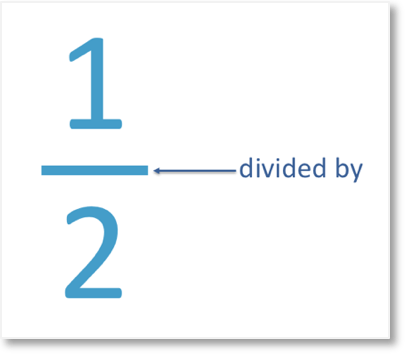The remainder is written as the numerator of the fraction and the denominator is the number we are dividing by.• 465 ÷ 2 is set out with the short division method.
• 4 ÷ 2 = 2 and so we write 2 above.
• 6 ÷ 2 = 3 and so we write 3 above.
• 5 ÷ 2 = 2 remainder 1.
• This is because 2 goes into 5 twice with 1 left over to get from 4 to 5.
• The remainder of 1 is now divided by 2 as well.
• We write 1 ÷ 2 as a fraction as  1 / 2
• 465 ÷ 2 = 132  1 / 2Supporting Lessons# Division with Remainders Written as Fractions

## Remainders as Fractions

A remainder is the number left over after a division. It has not been divided.

To write a remainder as a fraction, divide it by the number being divided by. The remainder becomes the numerator, on top of the fraction and the number being divided by becomes the denominator on the bottom of the fraction.

In this example below, we have 5 ÷ 2. We are sharing 5 carrots between 2 people.

We can give each person 2 carrots with one left over as a remainder.

We can write 5 ÷ 2 = 2 r1.However we can divide this remainder by 2 and give half to each person. They each have 2   1 / 2  .

We can write the remainder of 1 as a fraction.

5 ÷ 2 = 2   1 / 2  .

The remainder was 1 and we were dividing by 2. We wrote the remainder of 1 on top of the fraction and the 2 on the bottom of the fraction.The horizontal line that separates the numbers in a fraction is a division.

So, the fraction 12 means 1 divided by 2.

## Short Division Method with Remainders as Fractions

Here is an example of using the short division method. We have 465 ÷ 2.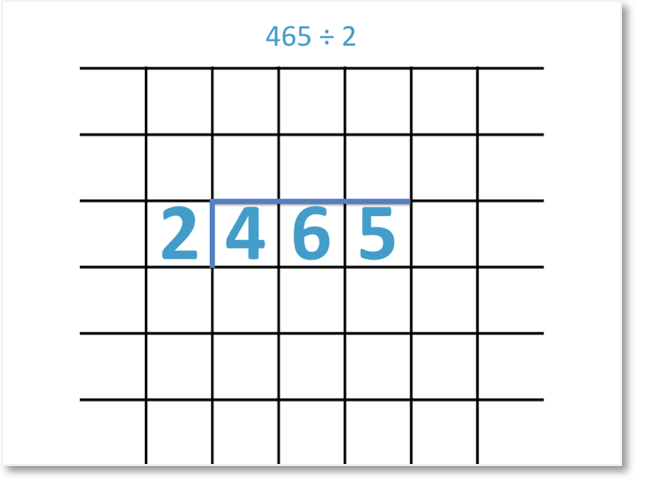We set out the short division calculation as shown above. We want to divide each digit in 465 by 2.

We work from left to right, starting with the 4.

4 ÷ 2 = 2

We write the 2 above the 4.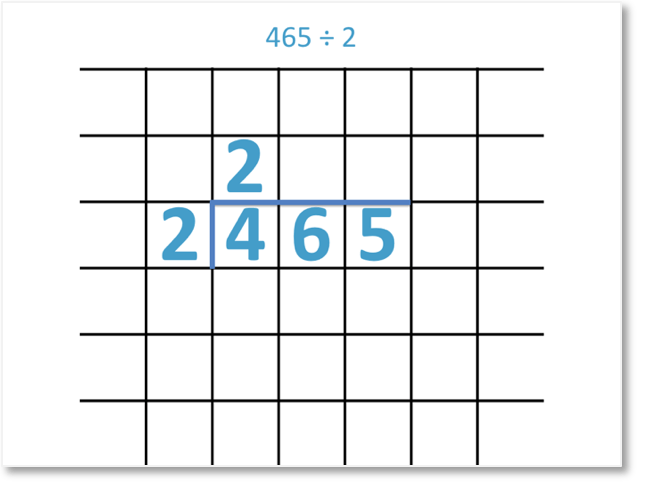6 ÷ 2 = 3

We write the 3 above the 6 in our division.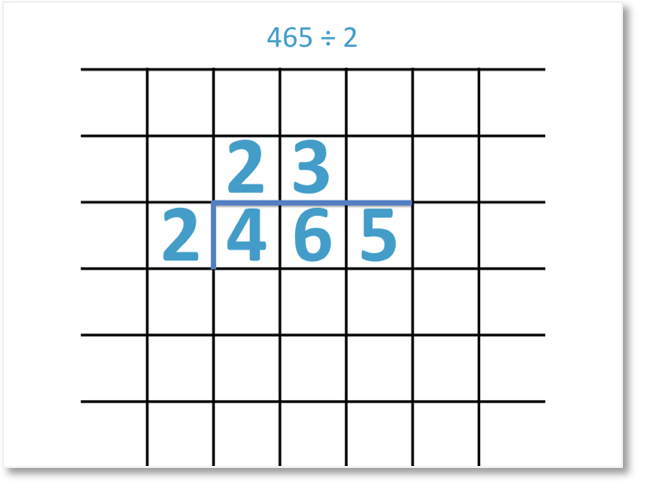2 divides into 5 twice, with 1 left over.

We write the 2 above the 5. There is a remainder of 1.We are going to write this remainder as a fraction.

To write a remainder as a fraction it is written as the numerator, with the number we are dividing by written below it as the denominator.

We have   1 / 2  .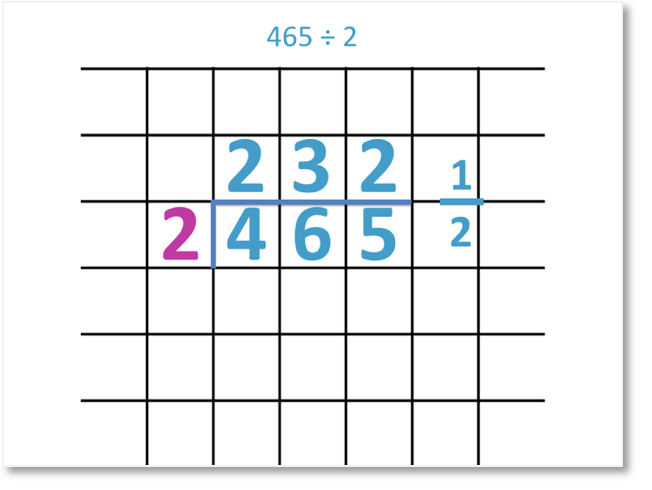Therefore,

465 ÷ 2 = 232   1 / 2  .Here is another example of using the short division method, writing the remainder as a fraction:

We have 517 ÷ 3.We set out the short division calculation as shown above. We want to divide each digit in 517 by 3.

We work from left to right, starting with the 5.

3 divides into 5 once, with 2 left over.We write the 1 above the 5 and we carry the 2 to meet the next digit along.

We now have 21 ÷ 3.

21 ÷ 3 = 7

We write the 7 above the 21.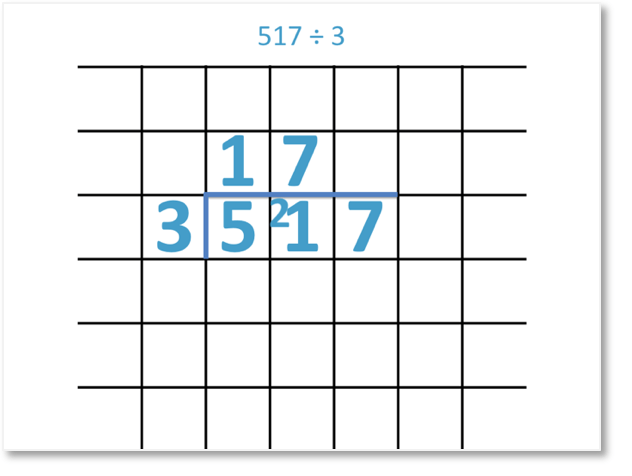3 divides into 7 twice, with 1 left over. We write the 2 above the 7.We have a remainder of 1, which we want to write as a fraction.

We divide the 1 by 3.

The fraction is   1 / 3   .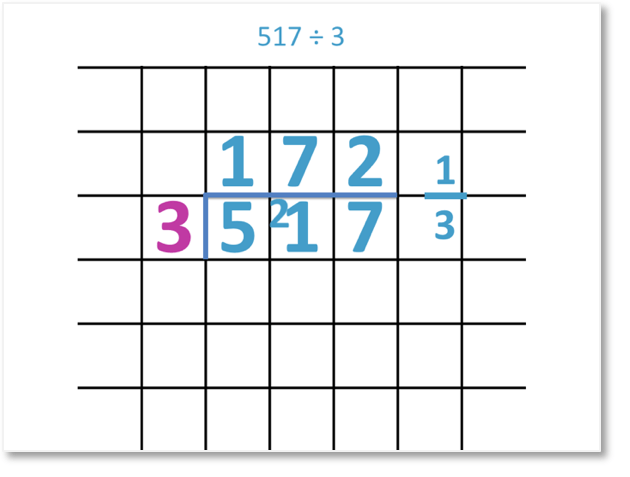Therefore,

517 ÷ 3 = 172   13  .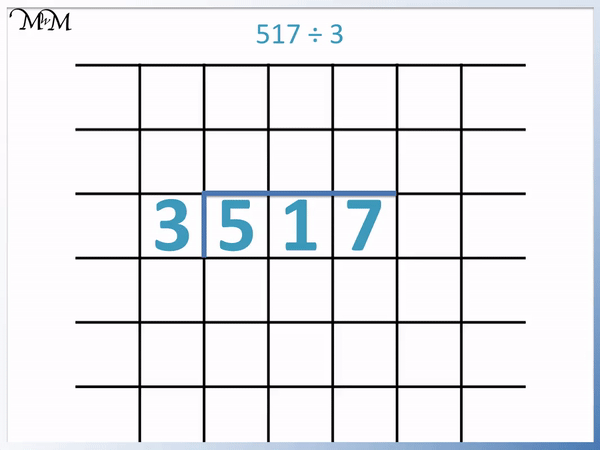In this next example we have 854 ÷ 4.

4 divides exactly twice into 8 and so, we write a 2 above 8.

5 ÷ 4 = 1 r1 so we carry the remainder of 1 over to meet the 4.

14 ÷ 4 = 3 remainder 2. This is because 4 times 3 equals 12.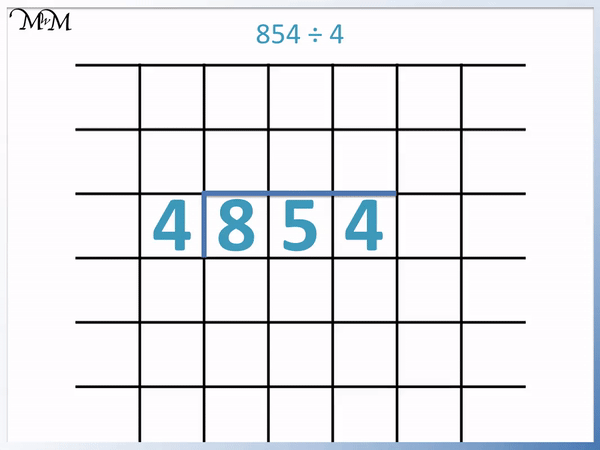We divide this remainder of 2 by 4 and write it as a fraction as   2 / 4   .

2 / 4   can be simplified to   1 / 2   because 2 is half of 4; .

854 ÷ 4 = 213 r   1 / 2   .

In this next example of short division with fractional remainders we have 789 ÷ 5.

7 ÷ 5 = 1 r2 and so we carry the 2 over to the 8 to make ’28’.

28 ÷ 5 = 5 r3 because 5 times 5 equals 25. We carry the remainder of 3 over to make 39.

39 ÷ 5 = 7 r4 because 5 times 7 = 35.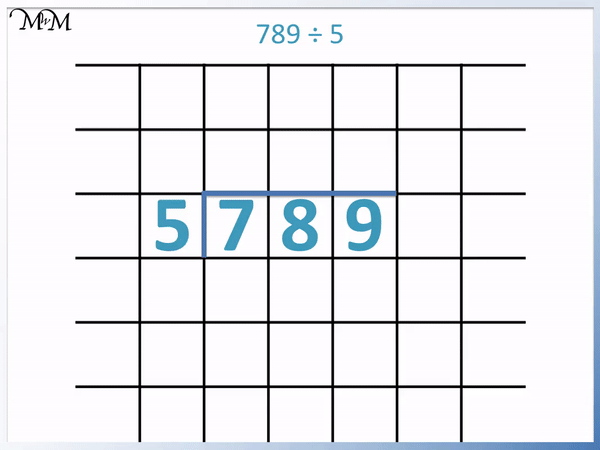The remainder of 4 is written as a fraction by also dividing it by 5. As a fraction it is written   4 / 5   .

And so 789 ÷ 5 = 157   4 / 5   .Now try our lesson on Short Division with Decimal Remainders where we learn how to write remainders as decimals.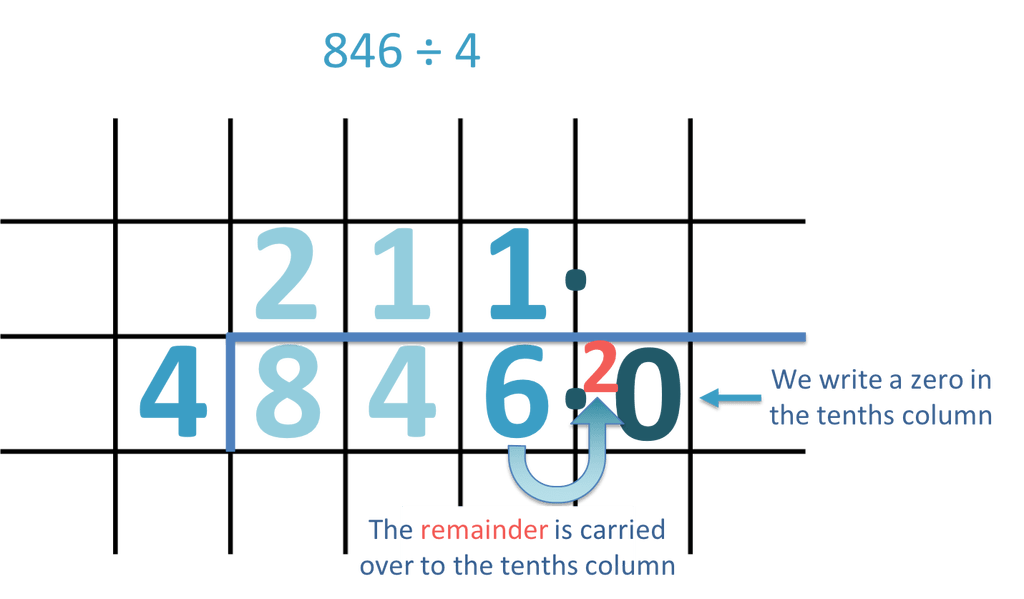error: Content is protected !!